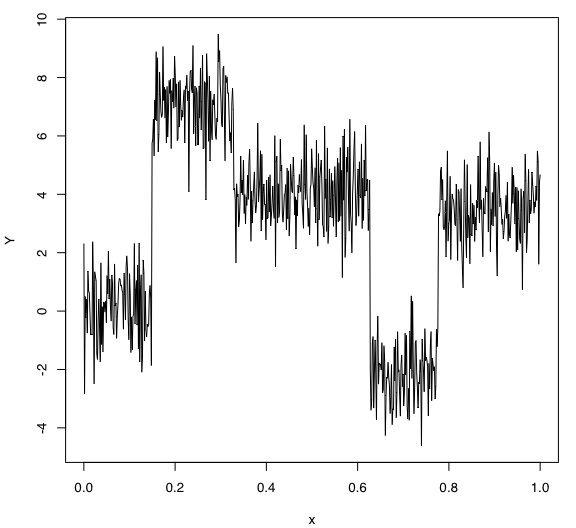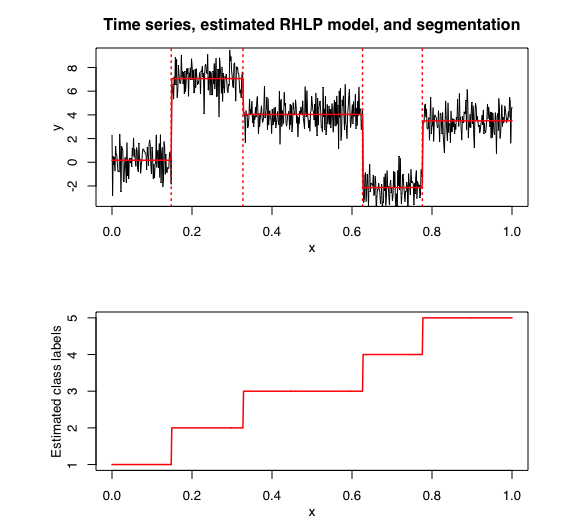# Introduction

In this package, it is possible to select models based on information criteria such as BIC, AIC and ICL.

The selection can be done for the two folliwng parameters:

• $$K$$: The number of regimes;
• $$p$$: The order of the polyniomial regression.

# Data

Let’s select a RHLP model for the following time series $$Y$$:

data("univtoydataset")
x <- univtoydataset$x y <- univtoydataset$y
plot(x, y, type = "l", xlab = "x", ylab = "Y")# Model selection with BIC

selectedrhlp <- selectRHLP(X = x, Y = y, Kmin = 2, Kmax = 6, pmin = 0, pmax = 3)
## The RHLP model selected via the "BIC" has K = 5 regimes
##  and the order of the polynomial regression is p = 0.
## BIC = -1041.40789532438
## AIC = -1000.84239591291

The selected model has $$K = 5$$ regimes and the order of the polynomial regression is $$p = 0$$. According to the way $$Y$$ has been generated, these parameters are what we expected.

Let’s summarize the selected model:

selectedrhlp$summary() ## --------------------- ## Fitted RHLP model ## --------------------- ## ## RHLP model with K = 5 components: ## ## log-likelihood nu AIC BIC ICL ## -982.8424 18 -1000.842 -1041.408 -1040.641 ## ## Clustering table (Number of observations in each regimes): ## ## 1 2 3 4 5 ## 100 120 200 100 150 ## ## Regression coefficients: ## ## Beta(K = 1) Beta(K = 2) Beta(K = 3) Beta(K = 4) Beta(K = 5) ## 1 0.1694561 7.06396 4.03646 -2.134881 3.495854 ## ## Variances: ## ## Sigma2(K = 1) Sigma2(K = 2) Sigma2(K = 3) Sigma2(K = 4) Sigma2(K = 5) ## 1.268475 1.125061 1.085376 1.011946 1.046146 selectedrhlp$plot(what = "estimatedsignal")